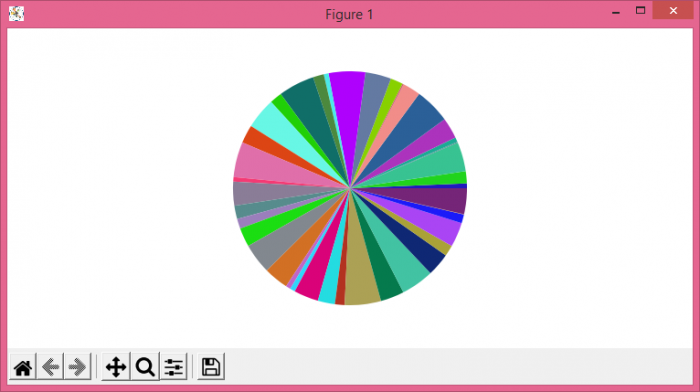# How can I generate more colors on a pie chart in Matplotlib?

To generate more colors on a pie chart in Matplotlib, we can generate n number of colors and data

## Steps

• Set the figure size and adjust the padding between and around the subplots.
• Initialize a variable, n, for number of data samples.
• Create random data points using numpy.
• Create a new figure or activate an existing figure.
• Add an '~.axes.Axes' to the figure as part of a subplot arrangement.
• Create a pie chart using pie() method.
• To display the figure, use show() method.

## Example

import matplotlib.pyplot as plt
import random
import numpy as np

plt.rcParams["figure.figsize"] = [7.50, 3.50]
plt.rcParams["figure.autolayout"] = True

n = 40
color = ["#" + ''.join([random.choice('0123456789ABCDEF')
for j in range(6)]) for i in range(n)]
a = np.random.random(n)
f = plt.figure()
plt.show()